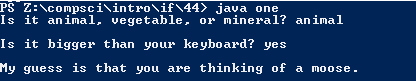# Assignemnt #44 - One

## Code

```    /// Name: Caelan Dorstad
/// Period: 6
/// Program Name: one
/// File Name: one.java
/// Date Finished: 10/19/2015

import java.util.Scanner;

public class one{
public static void main(String[] args){

Scanner keyboard = new Scanner(System.in);

String Q1, Q2, animal = "animal" , vegetable = "vegetable" , mineral = "mineral" , big = "yes", small = "no";

int R1=0, R2=0; // R1 = type R2 = Size

System.out.print("Is it animal, vegetable, or mineral? ");
Q1 = keyboard.next();
System.out.println();

if (Q1.equals(animal)) {
R1 += 1;
System.out.print("Is it bigger than your keyboard? ");
Q2 = keyboard.next();

if (Q2.equals(big)){
R2 += 1;
}

else if (Q2.equals(small)){
R2 += 2;
}
}

if (Q1.equals(vegetable)) {
R1 += 2;
System.out.print("Is it bigger than your keyboard? ");
Q2 = keyboard.next();

if (Q2.equals(big)){
R2 += 1;
}

else if (Q2.equals(small)){
R2 += 2;
}
}

if (Q1.equals(mineral)) {
R1 += 3;
System.out.print("Is it bigger than your keyboard? ");
Q2 = keyboard.next();

if (Q2.equals(big)){
R2 += 1;
}

else if (Q2.equals(small)){
R2 += 1;
}
}
System.out.println();

if ( R1 == 1 && R2 == 1) // animal & big
{
System.out.println("My guess is that you are thinking of a moose.");
}

else if ( R1 == 1 && R2 == 2) // animal & small
{
System.out.println("My guess is that you are thinking of a squirrel.");
}

else if ( R1 == 2 && R2 == 1) // plant & big
{
System.out.println("My guess is that you are thinking of a watermelon.");
}

else if ( R1 == 2 && R2 == 2) // plant & small
{
System.out.println("My guess is that you are thinking of a carrot.");
}

else if ( R1 == 3 && R2 == 1) // mineral & big
{
System.out.println("My guess is that you are thinking of Mustang 2015 V8!!!!!!");
}

else if ( R1 == 3 && R2 == 2) // mineral & small
{
System.out.println("My guess is that you are thinking of a paper clip.");
}

}
}
```

### Picture of the output